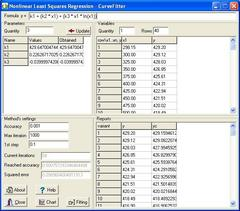Search
Software
Options for Regression Analysis - CurveFitter
Regression Analysis - CurveFitterCurveFitter program performs statistical regression analysis to estimate the values of parameters for linear, multivariate, polynomial, exponential and nonlinear functions. The regression analysis determines the values of the parameters that cause the function to best fit the observed data that you provide. This process is also called curve fitting.

Fit thousands of data into your equations in seconds:
Curvefitter gives scientists, researchers and engineers the power to find the ideal model for even the most complex data, including equations that might never have been considered. You can build equation set which can include a wide array of linear and nonlinear models for any application. Its state-of-the-art data fitting includes the following capabilities:

*Any user-defined equations of up to nine parameters and eight variables.
*Linear equations.
*Nonlinear exponential, logarithmic and power equations.
*A 38-digit precision math emulator for properly fitting high order polynomials and rationals.

Graphically Review Curve Fit Results:
Once your data have been fit, Curvefitter automatically sorts and plots the fitted equations by the statistical criteria of Standard Error. A residual graph as well as parameter output is generated for the selected fitted equation within the Review Curve Fit window.

With All This Power, it is Still Easy to Use:
Curvefitter takes full advantage of the Windows user interface to simplify every aspect of operation -- from data import to output of results. Import data from many popular file formats including Excel, Lotus and ASCII. Once your data are in the editor, create a custom equation set and start the automatic fitting process with a single mouse click. Curvefitter is highly intuitive, easy-to-use and remarkably simple to learn.